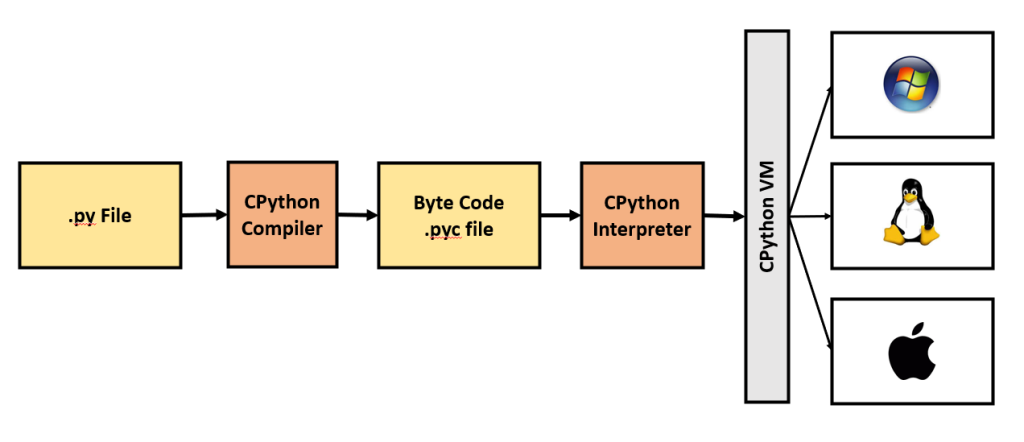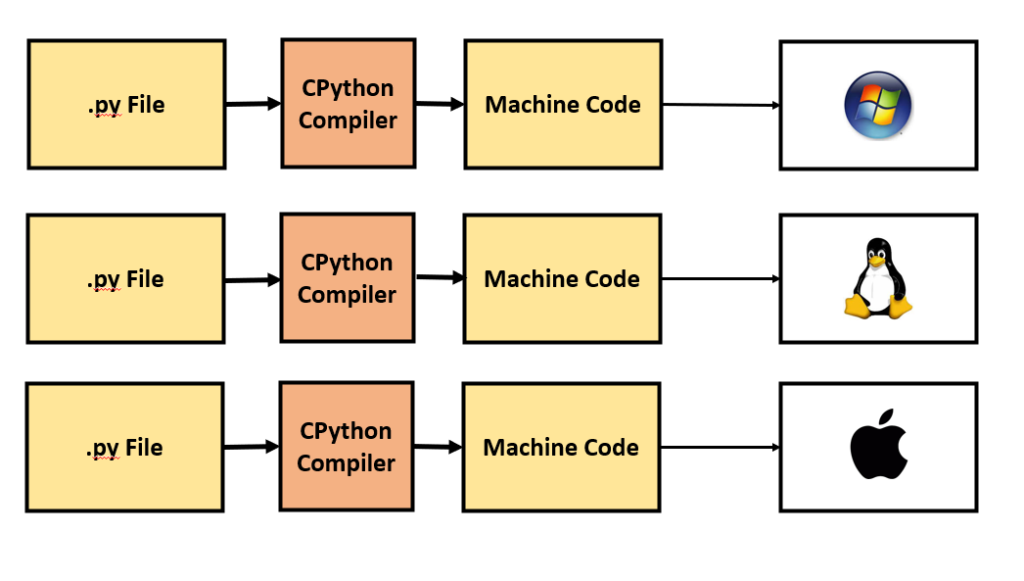# How to convert Python code to Cython (and speed up 100x)?

Using Cython, you can speed up existing Python code by an order of 100x or more. This is possible because Cython converts some of the Python code to C by doing some basic code changes.

Even without any code change a speed up of 2x is commonly observed, like in this post example.

Because, everything you write in Python is valid in Cython. And any Cythonizing code changes you make on top of that is only going to speed things up.In this post, we will see:

## 1. How does Cython make it run so fast?

Whenever you run a Python script, the following happens:

1. CPython compiles the source code (.py file) to generate bytecode (.pyc file).
2. CPython interpreter interprets the bytecode.
3. CPython virtual machine runs the output of the interpreter.

The CPython virtual machine helps make Python cross platform, but is time consuming. It makes Python slow.Cython provides the compiled machine level code specific to the operating system, that you directly run with without the need for a CPython interpret.Why does this make it so fast?

Python is dynamic in nature.

If you can define a variable as a number and then change it to text, python will not complain.

# Valid python code
a = 100
a = "some text"


However, Cython is statically typed. We define the type of the variable based on which ‘how much memory should be allocated to the datatype?” is decided.

This speeds up the code big time. You will see!

# Not valid
cdef int a = 100
a = "Some text"  # Raises ERROR!


## 2. Let’s write some python and measure running time

First let’s define the Python code we want to Cythonize and measure the code run time.

Step 1. Create a Python file (example.py) and place the following code.

def somefunc(K):
accum = 0
for i in range(K):
if i % 5:
accum = accum + i
return accum


Let’s measure how long this code takes to run.

import time
from example import somefunc

t1 = time.time()
somefunc(100_000_000)
t2 = time.time()
print(f"Time taken: {t2-t1} seconds")

Time taken: 5.129209280014038 seconds


## 3. How to Cythonize the Python script

Let’s now cythonize the Python script: 'example.py'.

Step 0. Install cython library

We will need the cython library, so let’s first install it by running the following in terminal or command prompt.

pip install cython


Step 1. Create the .pyx file

Place the contents of the python script (example.py) inside a .pyx file, say example_cy.pyx.

Alternately, if you don’t need the original python file, you can simply rename 'example.py' to 'example_cy.pyx'.

Here is the contents of 'example_cy.pyx':

def somefunc_cy(K):
accum = 0
for i in range(K):
if i % 5:
accum = accum + i
return accum


NOTE: We have done absolutely no change to the Python code, except changing the func name to somefunc_cy. This is only to distinguish which function is which.

All Python code is completely valid in Cython.

Step 2. Create a setup.py

Place the following code in setup.py and pass the path to the sample.pyx file (or the .pyx file you want to build).

from distutils.core import setup
from Cython.Build import cythonize
setup(
ext_modules = cythonize("example_cy.pyx")
)


In case, in future you have more than one script to Cythonize, you can place them all in a list and pass it to cythonize.

It might look something like this:

from distutils.core import setup
from Cython.Build import cythonize
setup(
ext_modules = cythonize(["script1.pyx",
"script2.pyx"])
)


Step 3. Run setup.py and build

Now, call setup.py from terminal or command prompt. If you

python3 setup.py build_ext --inplace


What happened just now?

On running this command, it will create a couple of files in the same directory – one with a .c extension and another with .pyd for windows or .so for linux.

This file is a compiled version of the python function which we can directly import and use.

Let’s now import the compiled function somefunc_cy and measure running time.

from example_cy import somefunc_cy

t1 = time.time()
somefunc_cy(100_000_000)
t2 = time.time()
print(f"Time taken: {t2-t1} seconds")

Time taken: 2.823068141937256 seconds


That’s a sweet 50% reduction in run time. We can further improve this by Cythonizing the code.

## 4. Cythonize a Python function by assigning C data types

There are essentially two things you need to take care of:

1. Define every variable using the “cdef” keyword and specify its data type.

For example: instead of a = 10, do cdef int a = 10. For very large variable, say variables where you accumulate values, you might use unsigned long long int.

2. Define every function to start with cpdef.

For example: def somefunc(K): will become cpdef somefunc(int K).

Here is the list of C data types and the range of values:Here is the updated contents of 'example_cy_static.pyx'.

cpdef unsigned long long int somefunc_cy2(long int K):
cdef unsigned long long int accum = 0
cdef long int i
for i in range(K):
if i % 5:
accum = accum + i
return accum


Then update the setup.py to include the new example_cy_static.pyx file.

from distutils.core import setup
from Cython.Build import cythonize
setup(
ext_modules = cythonize(["example_cy.pyx",
"example_cy_static.pyx"])
)


Then, compile again by running:

python3 setup.py build_ext --inplace


We can now import and call the somefunc_cy2 function.

from example_cy_static import somefunc_cy2

t1 = time.time()
somefunc_cy2(100_000_000)
t2 = time.time()
print(f"Time taken: {t2-t1} seconds")

Time taken: 0.14045310020446777 seconds


That is a brilliant improvement from 2.8 seconds to 0.14 seconds.

## 5. Optimize further to avoid unnecessary checks

We can add decorators that tells the compiler to avoid unnecessary checks such as ZeroDivisionError, NoneCheck etc.

By adding the following code, you can tell Cython to avoid doing the ZeroDivisionError check.

cimport cython
@cython.cdivision(True)


More on such directives here. Add more decorators exemptions as you see fit.

Here is the complete code, contents of example_cy_decor.pyx.

cimport cython

@cython.cdivision(True)
@cython.boundscheck(False)
@cython.wraparound(False)
@cython.nonecheck(False)
cpdef unsigned long long int somefunc_cy3(long int K):
cdef unsigned long long int accum = 0
cdef long int i
for i in range(K):
if i % 5:
accum = accum + i
return accum


Let’s import the function and run it.

from example_cy_decor import somefunc_cy3

t1 = time.time()
somefunc_cy3(100_000_000)
t2 = time.time()
print(f"Time taken: {t2-t1} seconds")

Time taken: 0.0852060317993164 seconds


We reduced the time taken further from 0.14 sec to 0.085 seconds. That is a significant gain.

So, overall we reduced the code run time from 5.1 seconds to 0.08 seconds using Cython. That’s massive!

I hope you now got the idea of how to Cythonize your own Python code.

## Similar Articles

### Logistic Regression – A Complete Tutorial With Examples in RCourse Preview

## Machine Learning A-Z™: Hands-On Python & R In Data Science

### Free Sample Videos:#### Machine Learning A-Z™: Hands-On Python & R In Data Science#### Machine Learning A-Z™: Hands-On Python & R In Data Science#### Machine Learning A-Z™: Hands-On Python & R In Data Science#### Machine Learning A-Z™: Hands-On Python & R In Data Science